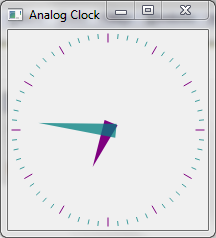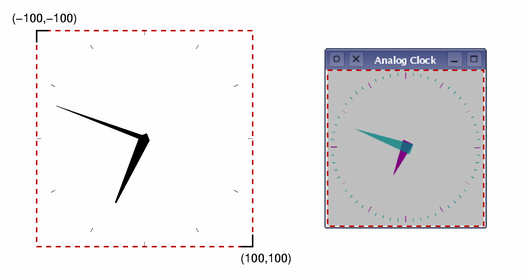# Analog Clock Window Example

The Analog Clock Window example shows how to draw the contents of a custom window.Clock Window example

This example demonstrates how the transformation and scaling features of QPainter can be used to make drawing easier.

## AnalogClockWindow Class Definition

The `AnalogClockWindow` class provides a clock with hour and minute hands that is automatically updated every few seconds. We make use of the RasterWindow from the Raster Window Example and reimplement the `render` function to draw the clock face:

```class AnalogClockWindow : public RasterWindow
{
public:
AnalogClockWindow();

protected:
void timerEvent(QTimerEvent *) override;
void render(QPainter *p) override;

private:
int m_timerId;
};```

## AnalogClock Class Implementation

```AnalogClockWindow::AnalogClockWindow()
{
setTitle("Analog Clock");
resize(200, 200);

m_timerId = startTimer(1000);
}```

We set a title on the window and resize to a reasonable size. Then we start a timer which we will use to redraw the clock every second.

```void AnalogClockWindow::timerEvent(QTimerEvent *event)
{
if (event->timerId() == m_timerId)
renderLater();
}```

The timerEvent function is called every second as a result of our startTimer call. Making use of the convenience in the base class, we schedule the window to be repainted.

Checking the timer's id is not strictly needed as we only have one active timer in this instance, but it is good practice to do so.

```void AnalogClockWindow::render(QPainter *p)
{
static const QPoint hourHand = {
QPoint(7, 8),
QPoint(-7, 8),
QPoint(0, -40)
};
static const QPoint minuteHand = {
QPoint(7, 8),
QPoint(-7, 8),
QPoint(0, -70)
};

QColor hourColor(127, 0, 127);
QColor minuteColor(0, 127, 127, 191);```

Before we set up the painter and draw the clock, we first define two lists of QPoints and two QColors that will be used for the hour and minute hands. The minute hand's color has an alpha component of 191, meaning that it's 75% opaque.

`    p->setRenderHint(QPainter::Antialiasing);`

We call QPainter::setRenderHint() with QPainter::Antialiasing to turn on antialiasing. This makes drawing of diagonal lines much smoother.

```    p->translate(width() / 2, height() / 2);

int side = qMin(width(), height());
p->scale(side / 200.0, side / 200.0);```

The translation moves the origin to the center of the window, and the scale operation ensures that the following drawing operations are scaled to fit within the window. We use a scale factor that let's us use x and y coordinates between -100 and 100, and that ensures that these lie within the length of the window's shortest side.

To make our code simpler, we will draw a fixed size clock face that will be positioned and scaled so that it lies in the center of the window.

We also determine the length of the window's shortest side so that we can fit the clock face inside the window.

The painter takes care of all the transformations made during the rendering, and ensures that everything is drawn correctly. Letting the painter handle transformations is often easier than performing manual calculations.We draw the hour hand first, using a formula that rotates the coordinate system counterclockwise by a number of degrees determined by the current hour and minute. This means that the hand will be shown rotated clockwise by the required amount.

```    p->setPen(Qt::NoPen);
p->setBrush(hourColor);```

We set the pen to be Qt::NoPen because we don't want any outline, and we use a solid brush with the color appropriate for displaying hours. Brushes are used when filling in polygons and other geometric shapes.

```    QTime time = QTime::currentTime();

p->save();
p->rotate(30.0 * ((time.hour() + time.minute() / 60.0)));
p->drawConvexPolygon(hourHand, 3);
p->restore();```

We save and restore the transformation matrix before and after the rotation because we want to place the minute hand without having to take into account any previous rotations.

```    p->setPen(hourColor);

for (int i = 0; i < 12; ++i) {
p->drawLine(88, 0, 96, 0);
p->rotate(30.0);
}```

We draw markers around the edge of the clock for each hour. We draw each marker then rotate the coordinate system so that the painter is ready for the next one.

```    p->setPen(Qt::NoPen);
p->setBrush(minuteColor);
p->save();
p->rotate(6.0 * (time.minute() + time.second() / 60.0));
p->drawConvexPolygon(minuteHand, 3);
p->restore();```

The minute hand is rotated in a similar way to the hour hand.

```    p->setPen(minuteColor);

for (int j = 0; j < 60; ++j) {
if ((j % 5) != 0)
p->drawLine(92, 0, 96, 0);
p->rotate(6.0);
}```

Again, we draw markers around the edge of the clock, but this time to indicate minutes. We skip multiples of 5 to avoid drawing minute markers on top of hour markers.

Files: